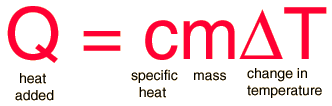# Specific Heat

The specific heat is the amount of heat per unit mass required to raise the temperature by one degree Celsius. The relationship between heat and temperature change is usually expressed in the form shown below where c is the specific heat. The relationship does not apply if a phase change is encountered, because the heat added or removed during a phase change does not change the temperature.The specific heat of water is 1 calorie/gram °C = 4.186 joule/gram °C which is higher than any other common substance. As a result, water plays a very important role in temperature regulation. The specific heat per gram for water is much higher than that for a metal, as described in the water-metal example. For most purposes, it is more meaningful to compare the molar specific heats of substances.

The molar specific heats of most solids at room temperature and above are nearly constant, in agreement with the Law of Dulong and Petit. At lower temperatures the specific heats drop as quantum processes become significant. The low temperature behavior is described by the Einstein-Debye model of specific heat.

 Table Specific heats of gases Specific heats of solids
 Calculation
Index

 HyperPhysics***** Thermodynamics R Nave
Go Back

# Specific Heat

The specific heat is the amount of heat per unit mass required to raise the temperature by one degree Celsius. The relationship between heat and temperature change is usually expressed in the form shown below where c is the specific heat. The relationship does not apply if a phase change is encountered, because the heat added or removed during a phase change does not change the temperature.Heat added = specific heat x mass x (tfinal - tinitial)

Enter the necessary data and then click on the active text above for the quantity you wish to calculate.

For a mass m = gm = kg

with specific heat c = cal/gm°C = joule/gm°C,

initial temperature Ti = °C = K = °F

and final temperature Tf = °C = K = °F,

the amount of heat added is

Q = calories = kcal = x 10^ calories.

Q = joules = x 10^ joules.

Calculation note: Unit conversions will be performed when you enter data, but the parameters will not be forced to be consistent until you click on the quantity you wish to calculate. Default values will be entered for unspecified parameters.

 Table Discussion of specific heat
Index

 HyperPhysics***** Thermodynamics R Nave
Go Back